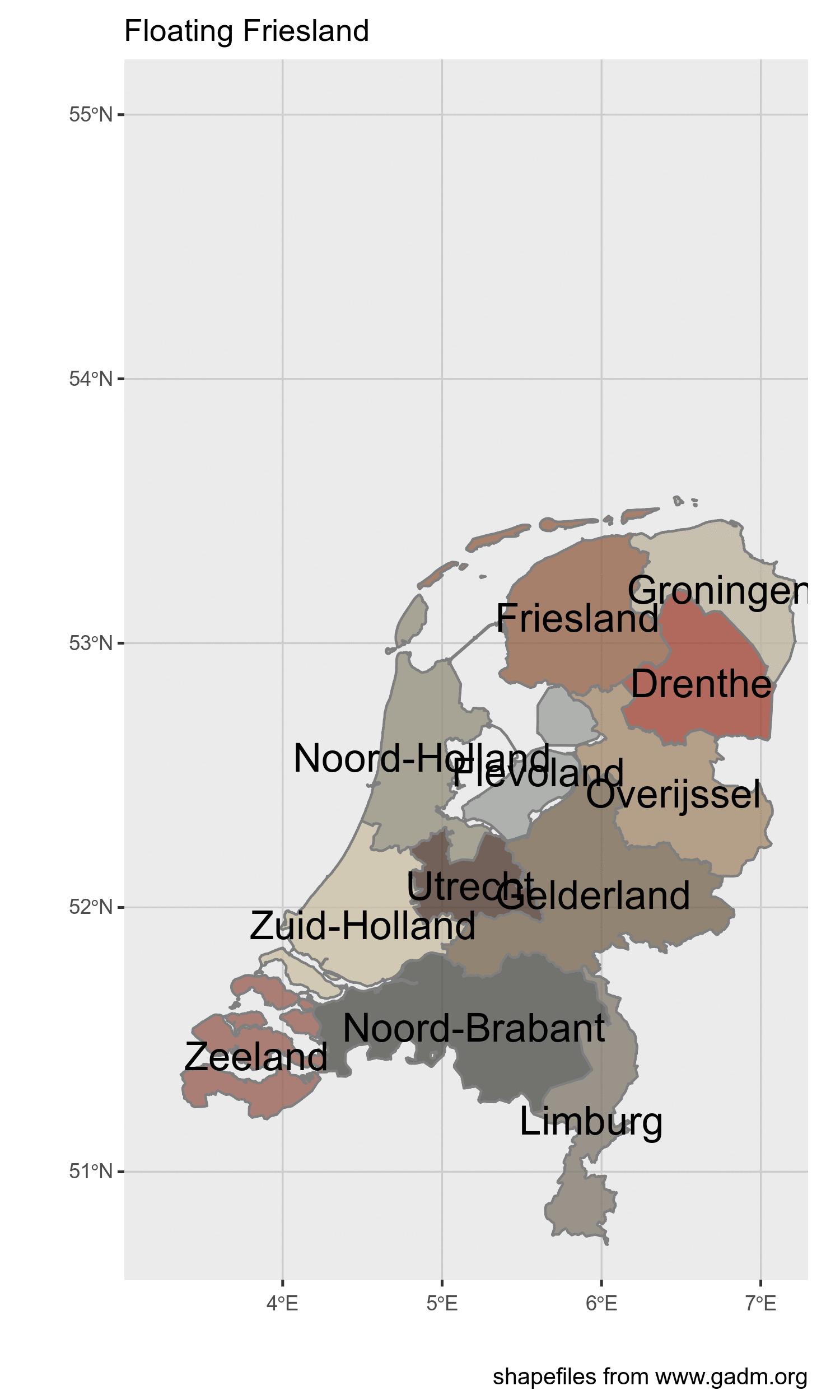# Moving parts of a country over a map

January 28, 2018
By

Want to share your content on R-bloggers? click here if you have a blog, or here if you don't.

I love making maps, I also love making gifs.
In this short post I make an animated gif of parts of a map moving. In this case the parts of the map only move in the xy direction, but you can also turn them, and make them bigger or smaller.
Today I show you how I made a part of the Netherlands ‘float away’. It is part of a larger nonsense project (I have many silly projects), and mostly just to document my path to learning about spatial analytics.

End result :## General principles

• make small functions that do one thing well (not that small in this case)
• combine those
• make imagemagick canvas
• write to the canvas
• animate the canvas

``````suppressMessages(library(tidyverse)) # ggplot2, dplyr, purrr, etc.
library(magick)
library(sf)
library(paletti) # thanks @edwinthoen

# colorscheme
dutchmasters_fill <- get_scale_fill(get_pal(dutchmasters))
# the data
``````

### basic functions

I created a function that takes a name, uses that to filter the data and apply a transformation on that part only. (a mutate_if() could also work, but I didn’t know how). And also one that uses that function to plot. The final function takes a matrix of xy values and sequentially applies every row to the plotting function.

• modify data
• plot a single ggplot version
• loop or apply over range
``````# basic function that moves an a province
move_province <- function(provincename, movement){
mov <- quo(movement)
rest <- NLD %>%
filter(NAME_1 != !!provincename) %>%
filter(TYPE_1 != "Water body")
#rest %>% st_centroid() %>% st_as_text()
province <- NLD %>%
filter(NAME_1 == !!provincename) %>%
mutate(geometry = geometry + !!mov) %>%
st_set_crs("+proj=longlat +datum=WGS84 +no_defs")

data1 <-
rbind(province, rest)
centroids <-
data1 %>% st_centroid()  %>% st_coordinates()
cbind(data1, centroids)
}
# make function to create plot
# using the previous function to move the province
plot_netherlands <- function(province, movement){
plotunit <- move_province(provincename = province, movement = movement) %>%
ggplot()+
geom_sf(aes(fill = NAME_1),color = "grey50", alpha = 3/4)+
geom_text(aes(X,Y, label = NAME_1), size = 6)+
lims(x = c(3.2,7.1), y = c(50.8,55))+
labs(x="", y = "", caption = "shapefiles from www.gadm.org", title = "Floating Friesland")+
dutchmasters_fill("little_street")+
theme( legend.position = "empty", # we already labeled the provinces
panel.grid.major = element_line(colour = "grey80"))
print(plotunit) # you have to explicitly tell it to print so the image is captured
}
# go over every frame and print
plot_province_over_range <-
function(offset_matrix, province = "Friesland", debug = FALSE){

if(any(is.na(offset_matrix))){stop("I cannot handle empty movements, there are NA's in movement_matrix")}
if(NCOL(offset_matrix) != 2) stop("movement_matrix needs to have exactly 2 columns")
actionsframe <- data_frame(x = offset_matrix[,1], y = offset_matrix[,2]) %>%
mutate(rownumber = row_number())
actionsframe\$name <- paste0(formatC(actionsframe\$rownumber, flag = 0,width = 4))

pb <- progress_estimated(NROW(actionsframe))

walk(actionsframe\$name, ~{

pb\$tick()\$print()
vars <- filter(actionsframe, name == .x)
if(debug){
message("using values from: ",vars)
}

plot_netherlands(province = province,movement = c(vars\$x[], vars\$y[]))

}) # ends the walk action
}
``````

### The plotting and saving

Nothing happened before the next step (except loading data). All the action and calculation happens here.

``````## then the creation starts with the movement
Friesland_moves <- rbind(
matrix(c(c(0,-.1,-.2,-.2,-.3), c(0,.03,.05,.1,.15)) ,ncol = 2),
matrix(c(seq(from = -.3, by = -.1, length.out = 14),seq(from = .2, by = .1, length.out = 14)), ncol = 2)
)
# set up print location
frames <- image_graph(width = 1500, height = 2500, res = 300, pointsize = 5)

plot_province_over_range(offset_matrix = Friesland_moves, province = "Friesland")

# animate
image_animate(frames, 1) %>%
image_write(path = "friesland.gif")
``````

I tried to edit this post on github on my mobile phone, boy, that does not work at all!

Moving parts of a country over a map was originally published by at Clean Code on January 29, 2018.

R-bloggers.com offers daily e-mail updates about R news and tutorials about learning R and many other topics. Click here if you're looking to post or find an R/data-science job.
Want to share your content on R-bloggers? click here if you have a blog, or here if you don't.